Principal and General Solutions

Chapter 3 Class 11 Trigonometric Functions
Serial order wise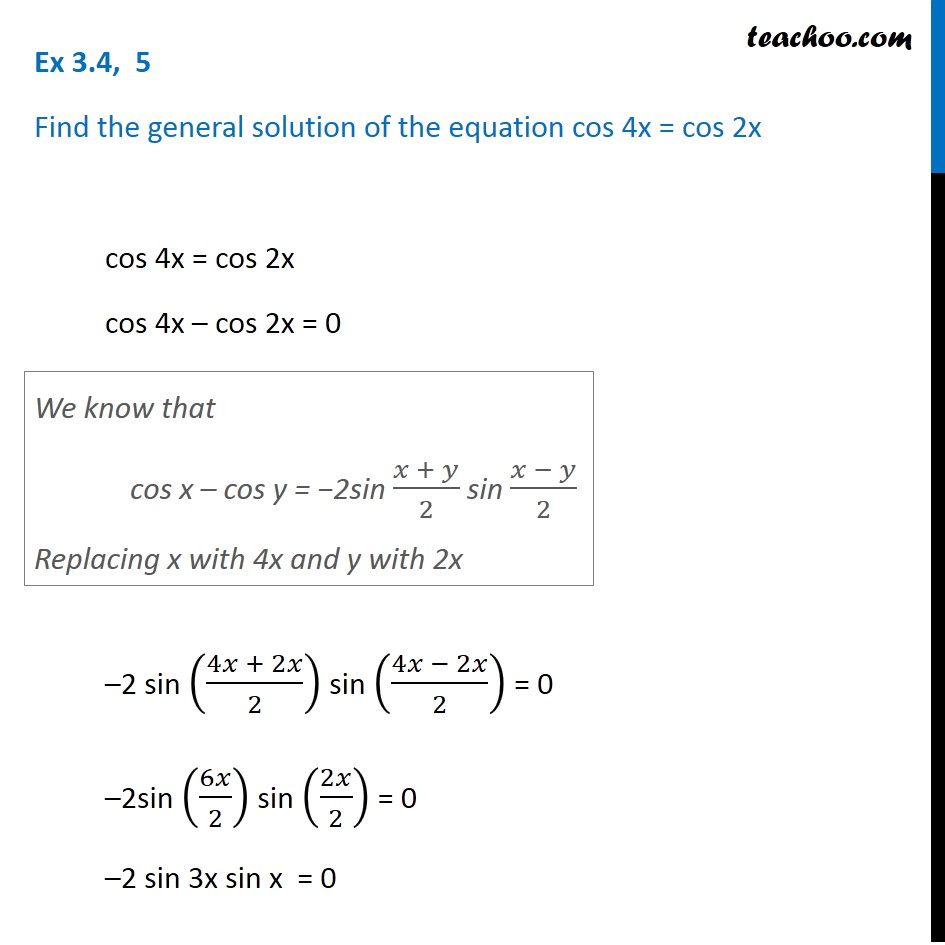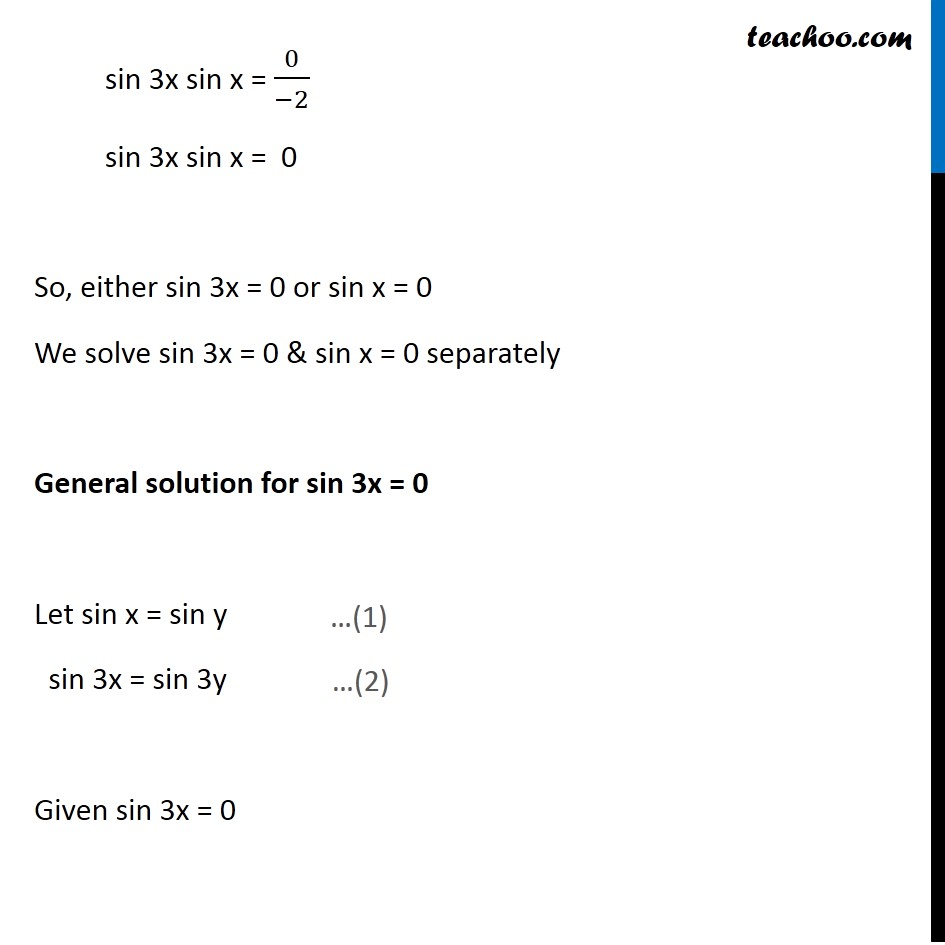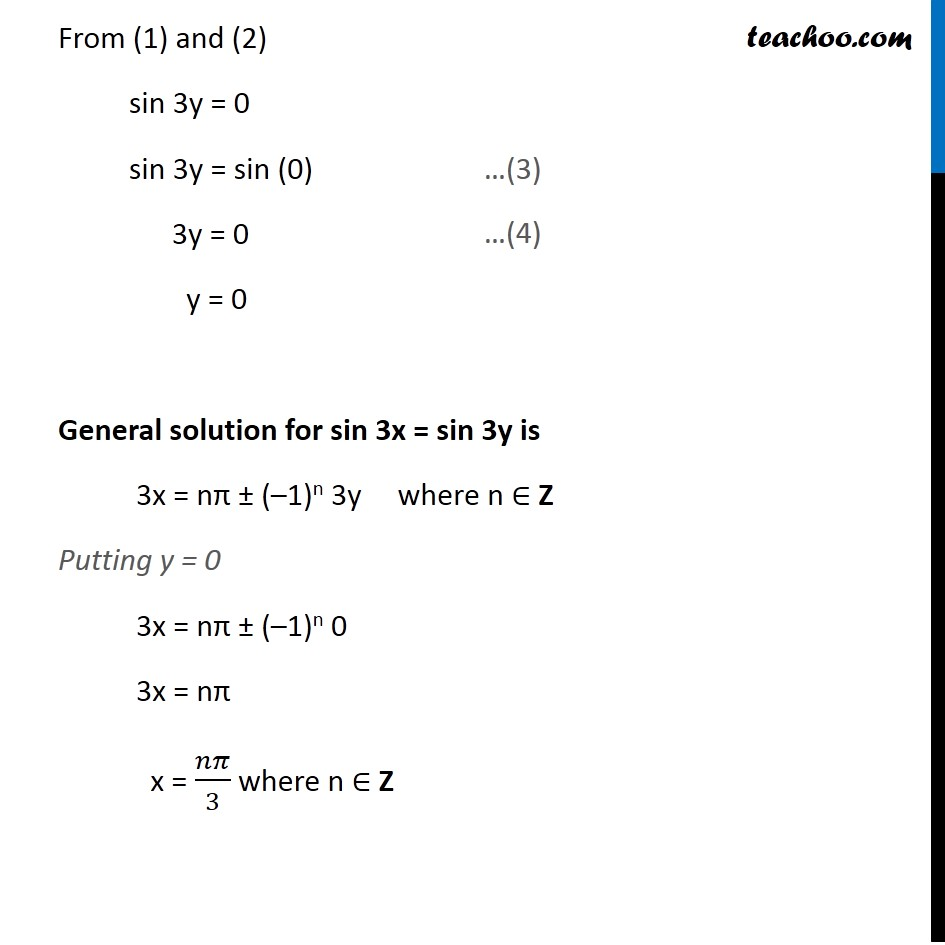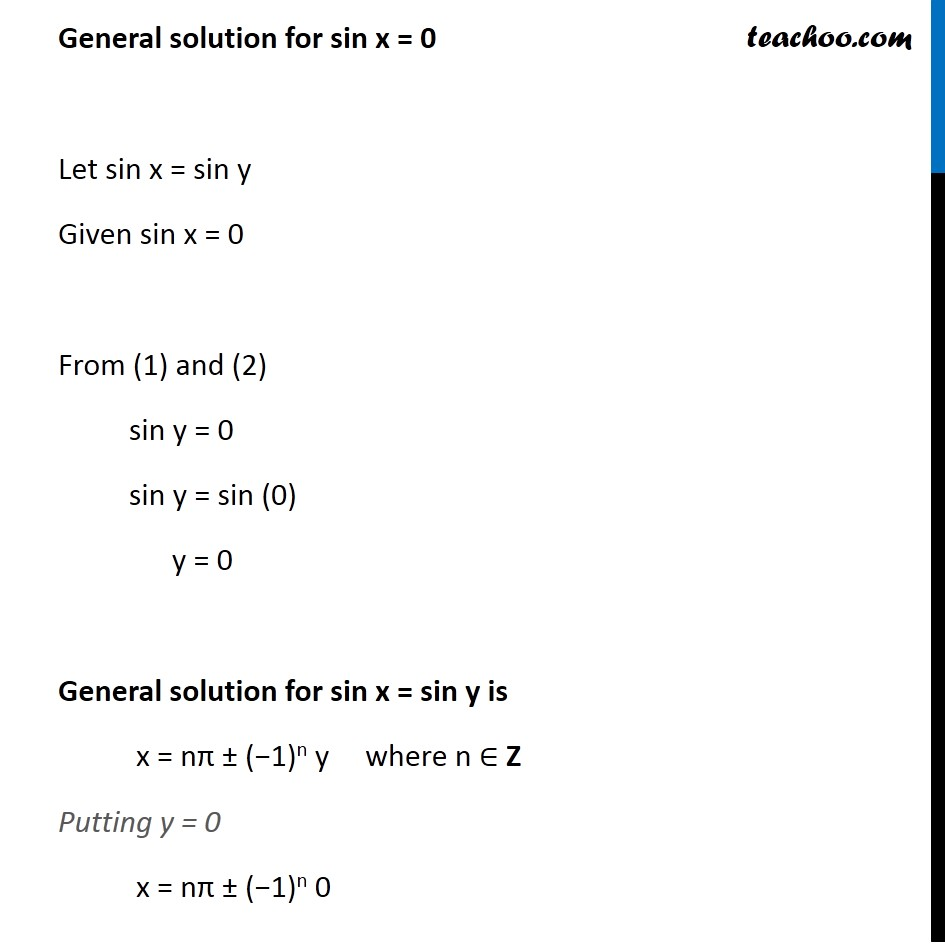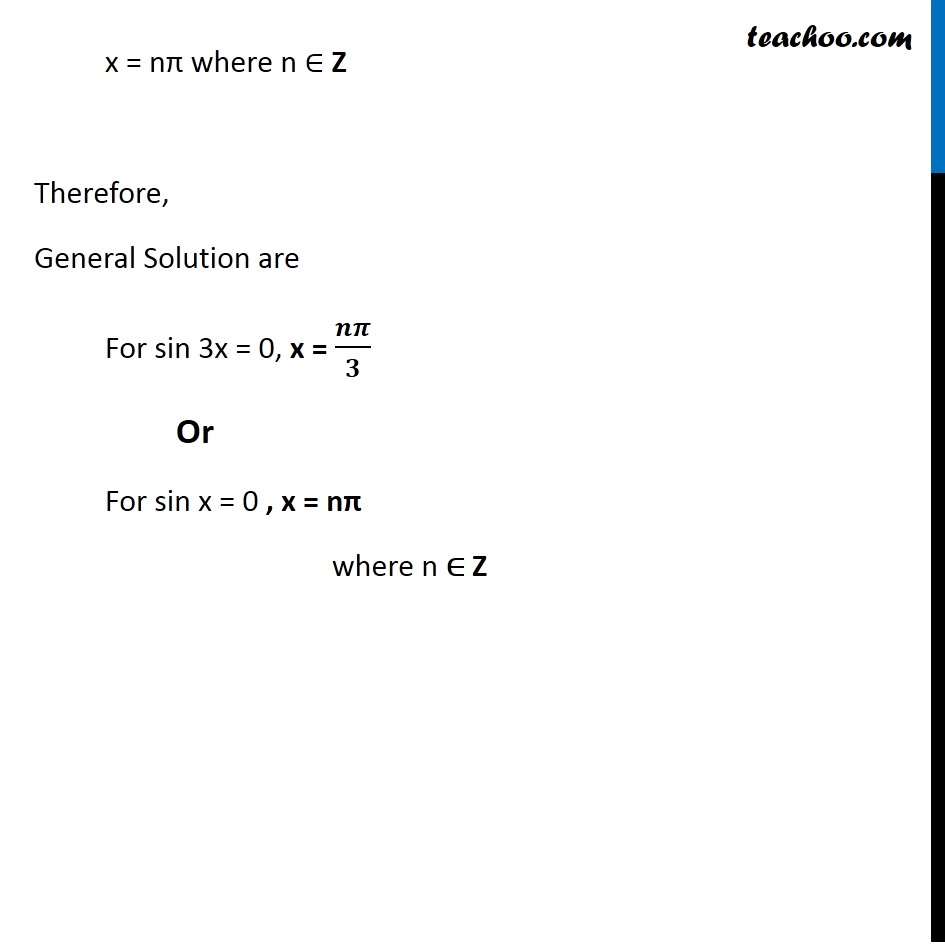Learn in your speed, with individual attention - Teachoo Maths 1-on-1 Class

### Transcript

Question 5 Find the general solution of the equation cos 4x = cos 2x cos 4x = cos 2x cos 4x – cos 2x = 0 –2 sin ((4𝑥 + 2𝑥)/2) sin ((4𝑥 − 2𝑥)/2) = 0 –2sin (6𝑥/2) sin (2𝑥/2) = 0 –2 sin 3x sin x = 0 We know that cos x – cos y = −2sin (𝑥 + 𝑦)/2 sin (𝑥 − 𝑦)/2 Replacing x with 4x and y with 2x sin 3x sin x = 0/(−2) sin 3x sin x = 0 So, either sin 3x = 0 or sin x = 0 We solve sin 3x = 0 & sin x = 0 separately General solution for sin 3x = 0 Let sin x = sin y sin 3x = sin 3y Given sin 3x = 0 From (1) and (2) sin 3y = 0 sin 3y = sin (0) 3y = 0 y = 0 General solution for sin 3x = sin 3y is 3x = nπ ± (–1)n 3y where n ∈ Z Putting y = 0 3x = nπ ± (–1)n 0 3x = nπ x = 𝑛𝜋/3 where n ∈ Z General solution for sin x = 0 Let sin x = sin y Given sin x = 0 From (1) and (2) sin y = 0 sin y = sin (0) y = 0 General solution for sin x = sin y is x = nπ ± (−1)n y where n ∈ Z Putting y = 0 x = nπ ± (−1)n 0 x = nπ where n ∈ Z Therefore, General Solution are For sin 3x = 0, x = 𝒏𝝅/𝟑 Or For sin x = 0 , x = nπ where n ∈ Z